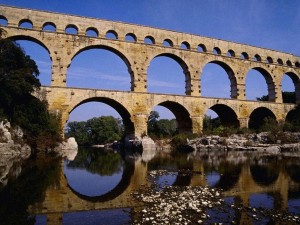# How did the Romans do the calculations necessary for construction and other purposes using Roman numerals?The Romans were skilled architects and engineers the likes of which the world had not seen before. They built aqueducts perfectly balanced so that water was carried entirely by gravity for up to 60 miles. They built huge elaborate and perfectly balanced structures that are not only still standing but still in use 2,000 years later. They were superb artisans and builders par excellence. However their number system, whilst useful, was anything but straight forward.

## Reading and Writing Roman Numerals

The big differences between Roman and Arabic numerals (the ones we use today) are that Romans didn’t have a symbol for zero, and that numeral placement within a number can sometimes indicate subtraction rather than addition.

Rather than use 9 digits the Roman numerals use letters each standing for a different number :

NumeralNumber
I1
V5
X10
L50
C100
D500
M1000

A string of letters means that their values should be added together. For example : XXX = 10 + 10 + 10 = 30, and LXI = 50 + 10 + 1 = 61. If a smaller value is placed before a larger one, we subtract instead of adding. For example : IV = 5 – 1 = 4.

You can use these rules to write a number in Roman numerals. Convert one digit at a time. Let’s try 982 :

982 = 900 + 80 + 2 = CM + LXXX + II = CMLXXXII

So how did the Romans manage to do the calculations necessary for construction and other purposes using Roman numerals using this rather unwieldy number system ?

## Doing the Math

Okay, can you imagine dividing CXVII by IX and carrying down numbers that look more like a cryptogram than an arithmetic problem?

The Romans were saved that torture. They relied on the Chinese abacus, with pebbles as counters, to perform their calculations. In fact mathematical operations were performed in Roman times by persons called ‘calculators’. They were so named because they used calcule (Latin for pebbles) to add, subtract, multiply and divide.

Author: Robert